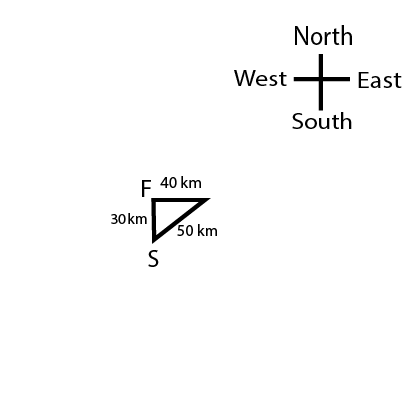0
173

# Sample Questions for RRB Group-D PDF

Download Top-15 RRB Group-D Sample Questions PDF. RRB GROUP-D Sample questions based on asked questions in previous exam papers very important for the Railway Group-D exam.

Question 1: Choose the alternative meaning of the bold part:
We should give a wide berth to bad characters

a) Publicity to

b) Publicly Condemn

c) Keep away from

d) Not sympathise with

Question 2: $\frac{2sinθ}{1+cosθ}$ = ?

a) 2cotθ/2

b) cotθ/2

c) tanθ/2

d) 2tanθ/2

Question 3: The soil salinity is measured by

a) Conductivity meter

b) Hygrometer

c) Psychrometer

d) Auxanometer

Question 4: Troposphere is the hottest part of the atmosphere because

a) it is closest to the Sun

b) there are charged particles in it

c) it is heated by the Earth’s surface

d) heat is generated in it

Question 5: Which one of the following does not belong to the group?

a) Monitor

b) Keyboard

c) Webcam

d) Mouse

Question 6: If it is Monday on 1st Jan 1991 then what day will it be on 1st Jan 1997?

a) Sunday

b) Monday

c) Saturday

d) Tuesday

Question 7: Arrange the following words in the order in which they appear in the dictionary:
1.Conjunctives
2.Contrast
3.Conducive
4.Conjure
5.Condemn

a) 5,3,1,4,2

b) 5,4,1,3,2

c) 5,3,4,1,2

d) 5,1,3,4,2

Question 8: East : North :: South : East :: North : ?

a) East

b) West

c) South

d) North

Question 9: If Sanju walks 50 km in north-east direction from a starting point and turns left to walk 40 km straight then how far is he from the starting point?

a) 40 km

b) 90 km

c) 35 km

d) 30 km

Question 10: Three of the following are similar in some way. The fourth one does not share the same characteristics as the other three. Choose the odd one out.

a) 273

b) 317

c) 307

d) 257

Question 11: Choose the odd term that is different from the other three:

a) Square

b) Pentagon

c) Hexagon

d) Octagon

Question 12: Which of the option figures bears the closest resemblance to the question figure?

a) A

b) C

c) B

d) D

Question 13: Out of the four option figures, three are similar in a certain manner. However, one figure is NOT like the other three. Select the figure which is different from the rest.

a) C

b) D

c) B

d) A

Question 14: If ‘+’ means ‘$\times$’, ‘-’ means ‘$\div$’, ‘$\times$’ means ‘-’, ‘$\div$’ means ‘+’, then find the value of $31+4\div3\times381-3$

a) -5

b) 0

c) 2

d) 1

Question 15: The characteristic of sound by which we can distinguish between two sounds of the same loudness and pitch is known as:

a) quality

b) intensity

c) pitch

d) loudness

“wide berth” means to keep a reasonable distance from someone.Thus, C is the right choice.

$\frac{2sinθ}{1+cosθ}$

= $\frac{2.2sinθ/2.cosθ/2}{2cos^2θ/2}$

= $\frac{2sinθ/2}{cosθ/2}$

= $2tanθ/2$

So the answer is option D.

Every year day gets ahead by 1 and after every leap year it will get ahead by 2 days. Hence, day will get ahead by 1+2+1+1+1+2 = 8 days. So answer will be Tuesday.

The first 3 letters are the same for all the five words.

Among condemn and conducive, condemn must precede since ‘d’ is followed by ‘e’.
After these 2 words, conjunction must follow them. Conjunction must be followed by conjure.
Contrast is the last term. Therefore, the correct order is condemn, conducive, conjunction, conjure and contrast. 5,3,1,4,2 is the correct order. Hence, option A is the right answer.

The second word is 90 degrees towards anti clockwise direction to the first word
=> 90 degrees anti clockwise of North is West.

The total journey line of Sanju will be along a right-angle triangle hence at last he will at a distance of $\sqrt{50^2 – 40^2} = 30$ km(Where S is starting point and F is final point)

317, 307 and 257 are prime numbers. They are not divisible by any number other than 1 and the number itself. 273 is a composite number. Hence, 273 is the odd one out.

Square, Hexagon and Octagon have even number of sides whereas Pentagon has odd number of sides.
Hence, pentagon is the one term.

After visualizing, We can see that option B is the most closest figure.

After decoding we have $31\times4+3-381\div3$